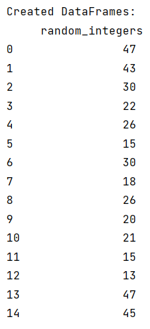# How to create a DataFrame of random integers with Pandas?

By using the random integers, we have to create a Pandas DataFrame. By Pranit Sharma Last updated : September 22, 2023

Pandas is a special tool that allows us to perform complex manipulations of data effectively and efficiently. Inside pandas, we mostly deal with a dataset in the form of DataFrame. DataFrames are 2-dimensional data structures in pandas. DataFrames consist of rows, columns, and the data.

## Creating a DataFrame of random integers with Pandas

To create a DataFrame of random integers in Pandas, we will use the random library of python. The random library is useful for generating random values within the provided range. The randint() method of the random library is used to generate random integers between the specified range.

Note

To work with pandas, we need to import pandas package first, below is the syntax:

```import pandas as pd
```

Let us understand with the help of an example,

## Python program to create a DataFrame of random integers

```# Importing pandas package
import pandas as pd

# Importing numpy package
import numpy as np

# Generating random integers
data = np.random.randint(10, 50, size=15)

# Creating a DataFrame
df = pd.DataFrame(data,columns=['random_integers'])

# Display DataFrame with NaN values
print("Created DataFrames:\n",df,"\n")
```

### Output

The output of the above program is: